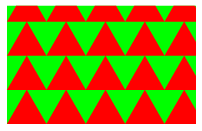Mathematical and Physical Journal
for High Schools
Issued by the MATFUND Foundation
 Already signed up? New to KöMaL?# Exercises and problems in InformaticsFebruary 2004

I. 70. Write an efficient program (i70.pas,...) that lists every integer between M and N (1$\displaystyle \le$M $\displaystyle \le$N $\displaystyle \le$10 000 000) whose number of divisors is a product of two primes.

(10 points)

I. 71. Prepare a program (i71.pas,...) that reads the side lengths of a rectangle and the side length of an equilateral triangle, then tiles the rectangle (from its bottom left corner) with rows of red triangles of the given size, and fills the remaining area inside with green.

The example shows a rectangle with height 300, width 480, and triangles with side length of 100.

(10 points)I. 72. Lucky numbers between the integers 1,2, ..., N can be found in the following way. To begin, strike out every second number, so we have 1, 3, 5, 7, 9, ... . The first number greater than 1 on this list is 3, so strike out every third number: we are left with 1, 3, 7, 9, 13, 15, 19, 21,... . Similarly, the first number on this list greater than 3 is 7, so strike out every seventh number resulting 1, 3, 7, 9, 13, 15, 21, ..., and so on. Having completed this sieve the remaining numbers are the lucky ones.

Prepare a sheet (i72.xls) so that writing the numbers 1, 2, 3, ..., N (2$\displaystyle \le$N $\displaystyle \le$100) into its first row, it displays the evolution of lucky numbers in the subsequent rows. Your solution should also work for any strictly monotone increasing sequence in the first row instead of 1, 2, 3, ..., N.

(10 points)

 1 2 3 4 5 6 7 8 9 10 11 12 13 14 15 16 17 18 19 20 21 1 3 5 7 9 11 13 15 17 19 21 1 3 7 9 13 15 19 21 1 3 7 9 13 15 21 1 3 7 9 13 15 21 1 3 7 9 13 15 21 1 3 7 9 13 15 21 1 3 7 9 13 15 21